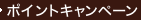•### Multi-Armed Bandit Allocation Indices (Wiley Interscience Series in Systems and Optimization)

• ただいまウェブストアではご注文を受け付けておりません。 ⇒古書を探す
• 製本 Hardcover:ハードカバー版／ページ数 256 p.
• 言語 ENG,ENG
• 商品コード 9780471920595
• DDC分類 519.5

### Full Description

Statisticians are familiar with bandit problems, operational researchers with scheduling problems, and economists with problems of resource allocation. Most such problems are computationally intractable and cannot be solved in polynomial time - which means that accurate solutions are unobtainable except for small-scale problems. This is particularly true under conditions of uncertainty. This book shows that there is, however, a large class of allocation problems for which the optimal solution is expressible in terms of a priority index which is defined for each of the competing projects independently of the properties of the other projects. Such problems are therefore solved once the appropriate index has been found. In some cases there is a concise formula for the index; at worst it can usually be determined by a manageable calculation. Since the discovery of the index, which has become known as the Gittins index, its properties and its range of applicability have been worked out in some detail. This book, which inaugurates a series in systems and optimization, gives an account of these developments and includes the first extensive tables of index values.

### Contents

Part 1 Main ideas: decision processes; bandit processes and simple families of alternative bandit processes; a first index theorem; jobs; the index theorem for jobs with no pre-emption; knapsacks; different discount functions; stochastic discounting; ongoing bandit processes; multiple processes. Part 2 Central theory: a necessary condition for an index; splicing bandit process portions; equivalent constant reward rates and forwards induction for arbitrary decision processes; more splicing and proof of the index theorem for a SFABP; near optimality of nearly index policies, and the gamma - O limit; bandit superprocesses and simple families of alternative superprocesses; the index theorem for superprocesses; stoppable bandit processes; the index theorem for a FABP with precedence constraints; precedence constraints forming an out-tree; FABPs with arrivals; minimum EWFT for the M/G/1 queue. Part 3 General properties of the indices: dependence on discount parameter; monotone indices; monotone jobs. Part 4 Jobs with continuously-varying effort allocations: competing research projects; continuous-time jobs; optimal policies for queues of jobs. Part 5 Multi-population random sampling (theory): jobs and targets; use of monotonicity properties; general methods of calculation - use of invariance properties; random sampling times; Brownian reward process; asymptotically normal reward processes. Part 6 Multi-population random sampling (calculations): normal reward process (known variance); normal reward process (mean and variance both unknown); Bernoulli reward process; exponential reward process; exponential target process; Bernoulli/exponential target process. Part 7 Search theory: a discrete search problem; two-person zero-sum games; a game of hide and seek; hide and seek in continuous time. Part 8 In conclusion: the Whittle index theorem; permutation schedules and sub-optimality; more about the Brownian reward process; more proofs, generalizations and extensions.